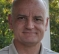## How to separate these orthogonal inputsStarted by 8 months ago12 replieslatest reply 7 months ago142 views

Hi All,

A receiver of ofdm separates orthogonal tones by using fft. No problem.

But there are some other signals that are also deemed orthogonal yet they are at same frequencies. Their orthogonality is by using phase.

For example SRS (in LTE) uses frequency sweep preamble (ZC sequences). some 8 users can share same one sequence but with cyclic shift and so transmit simultaneously.

How would receiver separate that. I imagine all 8 users will have same bin centre frequencies so FFT would not help. Then how does it work in order to separate them based on phase.

[ - ]Hi Kaz,

The cyclic shift (as defined actually) amounts to a time delay.
If you go to the time domain, and then window the specific "impulse" of interest, you can separate the individual components.
There are up to 3 different cyclic shift counts (6, 8, 12) depending on the comb factor.
I would be careful though.
Another issue I found is that a device may transmit from more than one antenna, and the individual antennae are supposed to use a different cyclic shift for each.
I found that for comb factor 2, and 2 antennae, there can destructive interference that nearly completely eliminates every other bin of the received signal of the SRS sequence.
If you have 2 antennae on the device that TX SRS, the cyclic shift (2 shifts used in this case) will put the one impulse at exactly half the symbol length compared to the other.  This is what causes the cancellation.

[ - ]Thanks David

Can you please elaborate on that window...in the following context:

Two UEs send same SRS signals on same REs. uplink radio receives them, does FFT and extracts SRS symbol(hence REs).

I can apply iFFT and so get to time domain which would be sum of two UE signals (with cyclic shift difference).

so what processing is needed from this point on.

Of course I can correlate with known references however I am not after peaks but rather after getting SRS signal across all REs from each UE in order to do channel estimation.

[ - ]I think is it is as simple as performing the IFFT on the combined received signal, selecting only the impulse response for the UE of interest, by zeroing out the other impulses, and then going back to the frequency domain.

You may choose to use some sort of window on the time domain after the selection process.

[ - ]Thanks David and dres,

Concluding from your replies and my analysis there seems to be two methods for SRS channel estimates (at least). A time domain based method that multiplies iFFT output by each channel cyclic shift and a frequency domain method that multiplies FFT output by conjugate of base preamble as mentioned in below paper:

I modelled the frequency domain method and it looks simpler.

[ - ]For LTE SRS, there are 8* possible cyclic shifts, which means the SRS are orthogonal over sub-lengths of 8 subcarriers. So for separating overlapping SRS's out for channel estimation, the best frequency resolution we can achieve is over a span of 8 subcarriers (which corresponds to 16 resource elements since the SRS are combed).

*For comb factor 2.

[ - ]I am afraid I haven't got what I was looking for...I am simply asking this:

I processed Uplink stream, got fft of each symbol, identified the SRS symbol, then separated it based on odd/even (for comb 2) and got say 24 samples of SRS shared by 8 UEs. How do I separate these 24 samples(subcarriers) among the 8 UEs if the 8 UEs have combined (added up in air having different cyclic shift).

Do you have any code or reference code to explain the processing.

Or put it in algebra terms: How come we add "A" to "B" with result "AB" received then extract back "A" and "B" knowing just they had different phases (orthogonal).

[ - ]Let's take this back to linear algebra basics. Suppose we have two orthonormal vectors x and y. Then we form the vector z = ax + by, where a and b are complex scalars. Then, I can recover a by doing a = dotprod(z,x) and I can recover b by doing b = dotprod(z,y).

Applying this to the problem at hand, x and y are SRS sequences with different cyclic shifts, while a and b are channel estimates.

[ - ]Thanks dres, that makes more sense.

So my question was wrong as I thought we are after recovering the original SRS preamble but as you confirmed we are after channel estimate.

[ - ]Here is another question that occurred to me related to this thread of the two types of orthogonality.

For ofdm the concept is explained on the basis that a peak of a tone (in frequency domain) coincides with a null of other subcarriers.

But what is the rational of cyclic shift orthogonality?

I checked both time domain and frequency domain of one preamble with cyclic shift but couldn't make any conclusion to explain how they don't interfere with each other.

[ - ]I think it stems from the autocorrelation property of ZC sequences.

The auto correlation of a Zadoff–Chu sequence with a cyclically shifted version of itself is zero, i.e., it is non-zero only at one instant which corresponds to the cyclic shift.

[ - ]sorry you misunderstood cyclic shift and cyclic prefix.

[ - ]